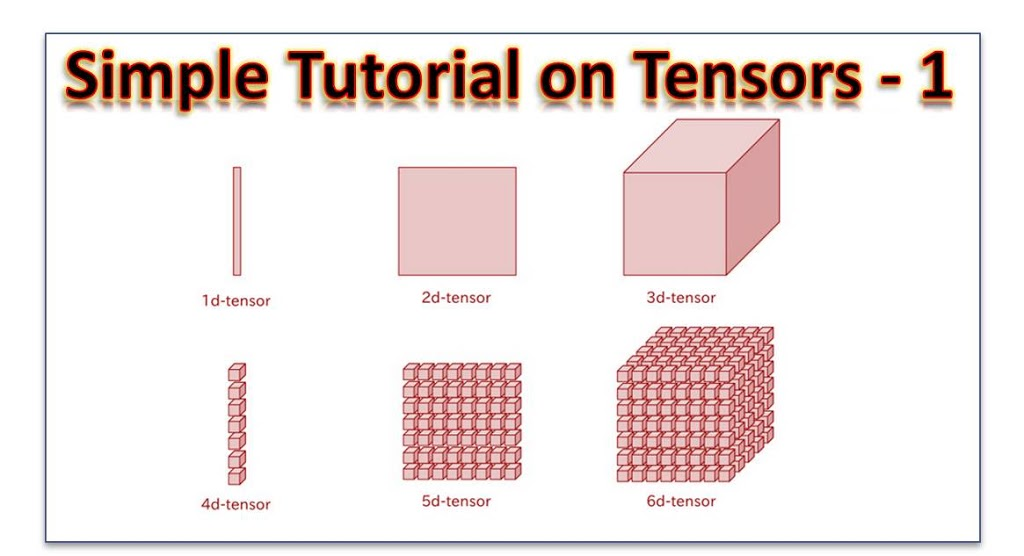# Simple Explanation of Tensors 1 – An IntroductionI have spent some time trying to understand tensors but I have spend more time trying to find an simple way to explain it to you.
The fact remains that to understand tensors, you need to take some time to think. But now I would try to make it easy be breaking it down into different chunks of short tutorials.

What you are reading now is the very first part (Simple Explanation of Tensors 1 – An Introduction). So you have a good start. In this first part, we would cover the following:

### 1. What is a Tensor(A Simple Definition)

Tensors are a type of data structure used in machine learning to represent various kinds of objects including  scalars, vectors, arrays, matrices and other tensors.
Some define tensors as multidimensional arrays. We would use this definition later, when we would be performing some tensor operation using Python and R.

### 2. Note About Representation

We would represent a scalar with a variable in square brackets. For example [a] is a scalar, not just a number.
We would represent the coordinate system using variable and subscripts. For example, the two dimiensional axes would be x1, x2 axis and not x, y. Similarly the 3 dimensional axis would be x1, x2, x3 axis and not x, y z.
The reason for this is that we can’t determine the limits of the dimensions of data using 26 letters alphabets.

This is illustrated in the figure:

To represent a scalar we just need just a single number [x1], e. g 15
To represent a vector we need two numbers [x1, x2] e.g [1, 3]
To represent an object in 3 dimension we need [x1, x2, x3] e.g [2, 3, 1]
Same in four dimension and so on

Now also note that a scalar is also a tensor of rank 1 (we would explain this later). A vector can be view as combination of two scalars. So a scalar is like a building block. (Remember from the definition of tensors that tensors are made up of other tensors.

### 3. Review of Vectors

A vector is normally viewed as a point in  space. For a two dimensional space  we would use two numbers. [2, 3]
For three dimensional space, we would use three numbers [3, 4, 2]
Vectors can also be represented as column of numbers.

What does the numbers represent?
The numbers does not represent distances along the the coordinate system. Rather, they represent direction. That is the endpoint coordinate the vector would reach if it were at the origin. This means that they don’t represent movement along the axis but direction in space.

### 4. Vector Transformation

Transformation refers to the changing of the coordinate system of a vector. In this case, the numbers representing the vectors change.
We would consider a transformation from X to X’.
The coordinate system X’ has its origin at (5,0,0) of the first system X.
Assuming we have a vector [1,1,0]. This vector is at point (0,0,0) of the coordinate system X’.
If we transform the coordinates from X’ to X, what would happen to the vector [1,1,0]?
What would happen is that the it would remain the same [1,1,0]. It does not change, just the coordinates of its position changes.

Why so?
Simply because, we did not move the vector! You only transformed the coordinate. The vector remains where it  is! Sure this is fair enough.

### 5. Representing Transformation

Let’s represent the transformation of the coordinate with T and  let’s call the vector V.
Let the transformation from X to X’ be called T’
Let the the transformation from X’ to X be called T.
Let’s call the vector V’ after transformation from X to X’

So we can write all of this in notations as:

• V’ = T'(V)
• V = T(V’)

You can read this, right?

What have all of this got to do with Tensors?
This is to let you know that there are transformation rules that applies to vectors.
We have played around with scalars and tensors. So I would like to state the following for you

• Scalars are tensors of rank
• Vectors are tensors of rank 1

Then comes the big question.
What the heck is a rank? We would continue this in Tutorial 2.

Note: If you have any challenge following, let me know in the comment box below or to the left of this page.